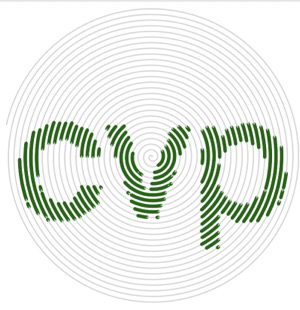# A program that draws a spiral with the user’s name in color (using the turtle module) browsing

• posted
0

I have written a little program that let’s the user enter his/her name and then draws a spiral with the name in four colors. The code works fine, but the names are not in color. I have reviewed it many times and I cannot find an error. I wonder, if the turtle module has the full functionality in Pythonista and if there are some restrictions.

Is there anybody that has some experience with the turtle module in Pythonista? Does the code seem incorrect to you? What would you change, that the names are printed in color?

Here is my code:

``````# SpiralMyName.py - prints a colorful spiral of the user's name

import turtle
t = turtle.Pen()
turtle.bgcolor('white')
colors = ['red', 'yellow', 'blue', 'green']

# Ask the user's name using turtle's textinput pop-up window
your_name = turtle.textinput('Enter your name', 'What is your name?')

# Draw a spiral of the name on the screen, written 100 times
for x in range(100):
t.penup() # Don't draw the regular spiral lines
t.forward(x*4) # Just move the turtle on the screen
t.pencolor(colors[x%4]) # Rotate through the four colors.
t.pendown() # Write the user's name bigger each time.
t.write(your_name, font = ('Arial', int((x+4)/4), 'bold'))
t.left(92) # Turn left, just as in our other spirals.
``````

• posted
1

• posted
1

@trezor please see here

• posted
0

Thanks @ccc and @cvp. Seems to be impossible in Pythonista, or at least not the way I want it to do. Thanks for the customized turtle documentation!

• posted
0

``````import turtle
import ui

# copy from standard module turtle.py
def write(self,text, move=False, align=None, font=None):
text = str(text)
w, h = ui.measure_string(text)
color = self._current_color
pos = self._pos
def _draw():
#ui.set_color(color)
# ui.draw_string uses its own key parameter color=
ui.draw_string(text, (pos.x, pos.y - h, 0, 0),color=color)
if move:
self._pos += (w, 0)
self.update_view()

turtle.Pen.write = write

# SpiralMyName.py - prints a colorful spiral of the user's name

t = turtle.Pen()
turtle.bgcolor('white')
colors = ['red', 'yellow', 'blue', 'green']

# Ask the user's name using turtle's textinput pop-up window
your_name = turtle.textinput('Enter your name', 'What is your name?')

# Draw a spiral of the name on the screen, written 100 times
for x in range(100):
t.penup() # Don't draw the regular spiral lines
t.forward(x*4) # Just move the turtle on the screen
t.pencolor(colors[x%4]) # Rotate through the four colors.
t.pendown() # Write the user's name bigger each time.
t.write(your_name, font = ('Arial', int((x+4)/4), 'bold'))
t.left(92) # Turn left, just as in our other spirals.
``````

• posted
0

@trezor, this is what your topic heading made me think you wanted.The code turned out to be a bit longer:

``````import io
import math

from PIL import Image

import turtle
import ui

canvas_color = 'white'
text_color = 'darkgreen'
text_line_width = 3
bg_color = 'lightgrey'
bg_line_width = 1

turtle.hideturtle()
t = turtle.Pen()
t.hideturtle()
t.speed(0)
turtle.bgcolor(canvas_color)

your_name = turtle.textinput('Enter your name', '')

# Create x/y addressable matrix from the name

label = ui.Label(
text=your_name,
text_color='black',
alignment=ui.ALIGN_CENTER,
)
label.size_to_fit()
with ui.ImageContext(label.width, label.height) as ctx:
label.draw_snapshot()
img = ctx.get_image()
with io.BytesIO(img.to_png()) as fp:
pil_image = Image.open(fp)

# Ratio to map from turtle coords to name coords

pw, ph = pil_image.size
tw, th = 250, 250
if pw/ph > tw/th:
ratio = tw/pw
else:
ratio = th/ph

# Function to check if a point is on text or not

def is_point_text(x, y):
x = x
y = -y
x = int(x/ratio + pw/2)
y = int(y/ratio + ph/2)
try:
return img_array[x, y] > 0
except IndexError:
return False

# Generator for the points of an evenly-spaced Arkhimedian spiral

def spiral_points(arc=1, separation=5):
def polar_to_coords(r, phi):
return r * math.cos(phi), r * math.sin(phi)
r = arc
b = separation / (2 * math.pi)
phi = float(r) / b
while True:
yield polar_to_coords(r, phi)
phi += float(arc) / r
r = b * phi

point_generator = spiral_points()

# Create the spiral

for i in range(11000):
x, y = next(point_generator)
if is_point_text(x, y):
t.pencolor(text_color)
t.pensize(text_line_width)
else:
t.pencolor(bg_color)
t.pensize(bg_line_width)
t.setposition(x, y)
``````

• posted
0

@mikael nice and thanks for your present...but it is far from using turtle, isn't it'

PS in French, we say "le nom des fous s'écrit partout", I let you translate in English/Finnish

• posted
0

@cvp, I know just enough French to understand such important sentences. 😁

If by ”not using turtle” you mean it is not just turning and moving forward, that would be easy to change (you can turn `toward` a point). But even ”more turtle” would be to have an Arkhimedean spiral built with an algorithm that just knows about turns and forward moves, and I could not find that algorithm, and my math fails on coming up with one on my own.

If you mean using ui and PIL, then yeah, cannot immediately think of a way to do those with turtle... Except cheating and writing the name with turtle and some suitable blend mode.

• posted
0

@mikael I only mean that I did not change his script at all, only add an own write...

Internal error.

Oops! Looks like something went wrong!